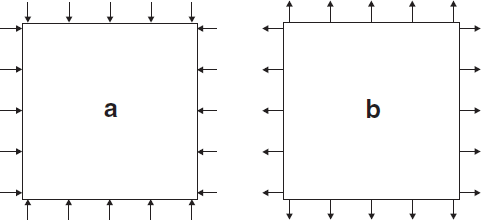# Sign Conventions

this page is based on the pfc version of the doc, with flac3d bits added to it.

The following sign conventions must be kept in mind when entering input or evaluating results.

DIRECT STRESS
Positive stresses indicate tension; negative stresses indicate compression.
SHEAR STRESS

With reference to Figure 1, a positive shear stress points in the positive direction of the coordinate axis of the second subscript if it acts on a surface with an outward normal in the positive direction. Conversely, if the outward normal of the surface is in the negative direction, then the positive shear stress points in the negative direction of the coordinate axis of the second subscript. The stresses shown in Figure 1 are all positive. The stress tensor is symmetric (i.e., complementary shear stresses are equal).Figure 1: Sign convention for positive stress components.

The distortion associated with positive and negative shear strain is illustrated in Figure 2. Stresses can be obtained from measurement spheres in PFC or from zones in FLAC3D.Figure 2: Distortion associated with positive and negative shear strain.

STRAIN RATE
The convention for strain rate is analogous to that for stress. Strain rate can be obtained from measurement spheres in PFC or from zones in FLAC3D.
CONTACT FORCE
Each contact-force vector can be resolved into normal and shear components with respect to the contact plane (see the “Contact Resolution” section for further details). Normal contact forces act normal to the contact plane, while shear contact forces act in the contact plane. Positive normal contact forces indicate compression; negative normal contact forces indicate tension.
CONTACT STRESS
The contact force is divided by the area associated with the contact. The sign convention is the same as for the contact force.
VECTOR QUANTITIES
The $$x$$-, $$y$$-, and $$z$$-components of all vector quantities (e.g., forces, moments, displacements, and velocities) are positive when pointing in the directions of the positive global $$x$$-, $$y$$-, and $$z$$-coordinate space.